Related Articles

# PySpark – Select Columns From DataFrame

• Last Updated : 04 Aug, 2021

In this article, we will discuss how to select columns from the pyspark dataframe. To do this we will use the select() function.

Syntax: dataframe.select(parameter).show()

where,

• dataframe is the dataframe name
• parameter is the column(s) to be selected
• show() function is used to display the selected column

Let’s create a sample dataframe

## Python3

 `# importing module``import` `pyspark` `# importing sparksession from pyspark.sql module``from` `pyspark.sql ``import` `SparkSession` `# creating sparksession and giving an app name``spark ``=` `SparkSession.builder.appName(``'sparkdf'``).getOrCreate()` `# list  of students  data``data ``=` `[[``"1"``, ``"sravan"``, ``"vignan"``], [``"2"``, ``"ojaswi"``, ``"vvit"``],``        ``[``"3"``, ``"rohith"``, ``"vvit"``], [``"4"``, ``"sridevi"``, ``"vignan"``],``        ``[``"1"``, ``"sravan"``, ``"vignan"``], [``"5"``, ``"gnanesh"``, ``"iit"``]]` `# specify column names``columns ``=` `[``'student ID'``, ``'student NAME'``, ``'college'``]` `# creating a dataframe from the lists of data``dataframe ``=` `spark.createDataFrame(data, columns)` `print``(``"Actual data in dataframe"``)``# show dataframe``dataframe.show()`

Output: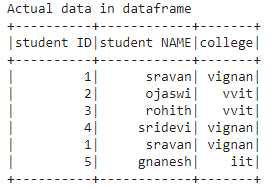### Selecting single Column

With column name, we can get the whole column in the data frame

Syntax: dataframe.select(“column_name”).show()

## Python3

 `# select column with column name``dataframe.select(``'student ID'``).show()`

Output: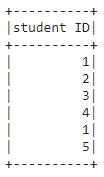### Selecting multiple Columns

With multiple column names, we can get the whole column in the data frame

Syntax: dataframe.select([“column_name1″,”column_name 2″,”column_name n”]).show()

## Python3

 `# select multiple column with column name``dataframe.select([``'student ID'``, ``'student NAME'``, ``'college'``]).show()`

Output: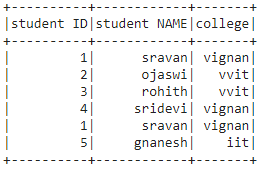### Selecting using Column Number

Here we are going to select the columns based on the column number. This can be done using the indexing operator. We can pass the column number as the index to dataframe.columns[].

Syntax: dataframe.select(dataframe.columns[column_number]).show()

## Python3

 `# select column with column number 1``dataframe.select(dataframe.columns[``1``]).show()`

Output: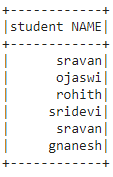Accessing multiple columns based on column number. Here we are going to select multiple columns by using the slice operator.

Syntax: dataframe.select(dataframe.columns[column_start:column_end]).show()

where, column_start is the starting index and column_end is the ending index

## Python3

 `# select column with column number slice``# operator``dataframe.select(dataframe.columns[``0``:``3``]).show()`

Output: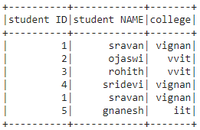Attention geek! Strengthen your foundations with the Python Programming Foundation Course and learn the basics.

To begin with, your interview preparations Enhance your Data Structures concepts with the Python DS Course. And to begin with your Machine Learning Journey, join the Machine Learning – Basic Level Course

My Personal Notes arrow_drop_up